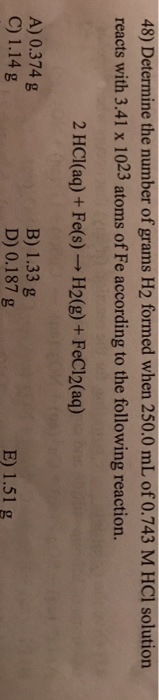# Question & Answer: Determine the number of grams H_2 formed when 250.0 mL of 0.743 M HCl solution reacts…..Determine the number of grams H_2 formed when 250.0 mL of 0.743 M HCl solution reacts with 3.41 times 10^23 atoms of Fe according to the following reaction. 2 HCl(aq) + Fe(s) rightarrow H_2(g) + FeCl_2(aq) A) 0.374 g B) 1.33 g C) 1.14 g D) 0.187 g E) 1.51 g

We know that

Mol=concentration*volume

Don't use plagiarized sources. Get Your Custom Essay on
Question & Answer: Determine the number of grams H_2 formed when 250.0 mL of 0.743 M HCl solution reacts…..
GET AN ESSAY WRITTEN FOR YOU FROM AS LOW AS \$13/PAGE

Mol of HCl=0.743*0.25=0.186 mol

Now

So mol of Fe=3.41*10^23/6.022*10^23=0.566 mol

Now according to stoichiometry,

2 mol of HCl=1 mol of Fe

So if we use limiting reactant as Fe than

We required 1.132 mol of HCl.but we have 0.186 mol of HCl.

So limiting reactant is HCl

So HCl mol=0.186

As per reaction

Fe required=0.0929 mol

So Fe in excess

So H2 mol=0.0929 mol

H2 Mass=mol*mol.weight=0.0929*2=0.186 gm